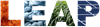WEAP is an initiative of the Stockholm Environment Institute. About WEAPHomeWhy WEAP?FeaturesWhat's New?Sample ScreensDemonstrationPublicationsHistory and Credits Using WEAPDownloadLicensingUser GuideTutorialVideos (YouTube) User ForumDiscussionsMembers ListEdit Profile Additional SupportTrainingUniversity CoursesCollaboration About UsSEI-US Water Resources ProgramPlease Contact UsInterested in Energy?Read about LEAP: SEI's software for energy planning.Link WEAP and LEAP for combined Water-Energy planning.Watch a video demo!

User Forum

 All Topics | Topic "GrowthAs function"

Author Message
Mr. Jack Sieber

Subject: GrowthAs function
Posted: 10/19/2005 Viewed: 36255 times
GrowthAs function Jack Sieber jsieber <a href="mailto:jsieber@tellus.org">jsieber@tellus.org</a>Reposting a question and answer about the WEAP GrowthAs function.

Question:

I would like to ask you about GrowthAs. How to use this expression? I read the User Guide but don’t understand. The example show only GDP case study, if we use to another way how to?

The GrowthAs function allows you to link the change in one variable to the change in another variable. For example, you might want to explore the relationship between the cost of water and the demand for water(in WEAP, this would be a change to the "water intensity" variable for a demand site). Perhaps as the cost doubles, the demand goes down by 25%. (This is an inverse relationship--as one increases, the other decreases--and will have an elasticity less than 0.) The expression for demand (the "water intensity" variable) will be

GrowthAs(\Key Assumptions\Price of Water, elasticity).

(This assumes that you had created a Key Assumption variable called Price of Water, and had specified the current and future values of the price. WEAP will not do this for you.)

To derive the elasticity, let's use our assumptions. NamedBranchValue doubles:

NamedBranchValue(t) = 2 * NamedBranchValue(t-1)

Current Value decreases by 25%:

Current Value(t) = 0.75 * Current Value(t-1)

Using the definition of GrowthAs with elasticity

Current Value(t) = Current Value(t-1) * ( (NamedBranchValue(t) / NamedBranchValue(t-1)) ^ Elasticity )

substitute our assumptions:

0.75 * Current Value(t-1) = Current Value(t-1) * ( (2 * NamedBranchValue(t-1) / NamedBranchValue(t-1)) ^ Elasticity )

Simplifying:

0.75 = 2 ^ Elasticity

Take the log base 2 of each side:

Elasticity = log2(0.75) = -0.415

Therefore, the expression to use for the water intensity would be GrowthAs(\Key Assumptions\Price of Water, -0.415)

In modeling demand, in addition to looking at the price elasticity of demand (demand decreases as price increases), you might also want to explore the income elasticity of demand (demand increases as income or GDP increases). For more information on elasticities, consult a basic economics textbook.

Jack
 Topic "GrowthAs function"